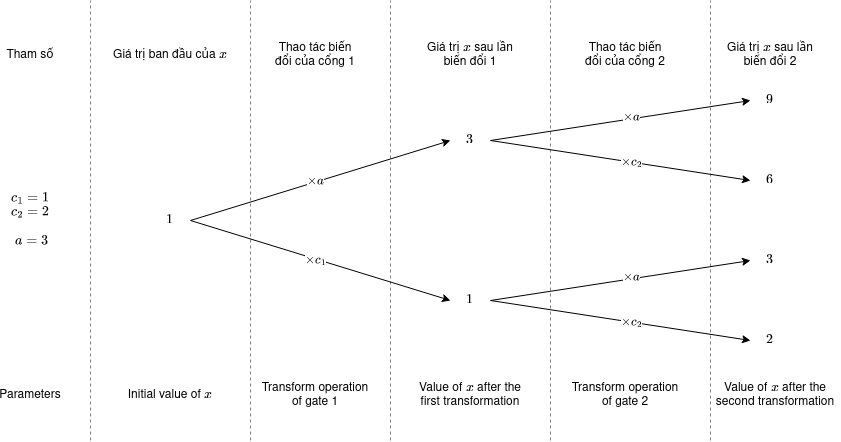## Quantum supercomputer

View as PDF

Points: 1.60 (partial)
Time limit: 4.0s
Memory limit: 256M
Input: stdin
Output: stdout

Problem type
Allowed languages
C, C++, Java, Kotlin, Pascal, PyPy, Python, Scratch

This is an interactive problem.

~\textit{ngfam}~ has a quantum supercomputer peculiarly structures with ~n~ quantum gates numbered from ~1~ to ~n~. The ~i~-th quantum gate has a characteristic parameter ~c_i~ ~(0 \leq c_i \leq 100)~.

To use the supercomputer, the user needs to input a non-negative integer ~a~. At the same time, the computer initializes a variable ~x = 1~. Afterwards, the quantum gates from ~1~ to ~n~ will be activated sequentially. When activating the ~i~-th gate, the computer changes ~x~ according to one of the following possibilities:

• ~x \leftarrow (x \times a) \bmod 998\,244\,353~

• ~x \leftarrow (x \times c_i) \bmod 998\,244\,353~

in which ~a \bmod b~ denoting the remainder of ~a~ when divided by ~b~.

Each outcome has the same probability of happening, and there are totally ~2^n~ outcomes in the activation process of all quantum gates. However, since this is a quantum supercomputer, so instead of returning a specific value of ~x~, it returns the overloaded value of ~x~, equivalent to the expected value of ~x~ for all possible scenarios.

As the creator of the supercomputer, ~\textit{ngfam}~ has initialized the ~c_i~ parameter of the ~n~ gates to his liking, but ~\textit{ngfam}~ won't be telling you this info. On the other hand, ~\textit{ngfam}~ will let you play with his computer ~2 \cdot n~ times: you can pick the parameters ~a~ you want, and the computer returns the corresponding ~x~. When you're done, ~\textit{ngfam}~ wants to make sure that you understand what's going on in his supercomputer, therefore you need to answer to ~\textit{ngfam}~ ~q~ of the same questions from ~\textit{ngfam}~ without using his computer this time.

Note that the overloaded value itself may not be an integer, but the computer will return said value modulo ~998\,244\,353~.

Formally, let ~M = 998\,244\,353~ and the overloaded value is an irreducible fraction ~\frac{p}{q}~, where ~p~ and ~q~ are integers and ~q \not \equiv 0 \pmod{M}~. The computer returns ~p \cdot q^{-1} \bmod M~. In other words, the computer returns such an integer ~r~ that ~0 \le r < M~ and ~r \cdot q \equiv p \pmod{M}~.

#### Input

The first line contains two integers ~n~ and ~q~ (~1\le n \le 50\,000~, ~1 \le q \le 50~) denoting the number of quantum gates, and the number of questions you should answer, respectively.

Afterwards you should interact with the jury program.

#### Interaction

You are allowed to pose a maximum of ~2 \cdot n~ questions, each question must be printed by the form ? a (~0 \leq a < 998\,244\,353~). The jury responds with a single integer, that is the overloaded value of ~x~ after using the value ~a~.

When you're done, output on a single line ! denoting you are ready to answer questions.

Afterwards, you have to answer ~q~ questions from ~\textit{ngfam}~. For each question, the jury program gives you a single integer ~a~ (~0 \le a \le 998\,244\,353~). You need to calculate and provide the overloaded value of ~x~ that the computer would've returned if supplied with said value ~a~. You have to answer the questions sequentially, meaning you won't receive the next question (if any) without answering the current one first.

After printing a query do not forget to output end of line and flush the output. Otherwise, you will get Time Limit Exceeded. To do this, use:

• fflush(stdout) or cout.flush() in C++;
• System.out.flush() in Java;
• flush(output) in Pascal;
• stdout.flush() in Python;
• see documentation for other languages.

#### Scoring

• Subtask 1 (~20~ points): ~n \le 50~ and ~1 \le c_i \le 2~.

• Subtask 2 (~60~ points): ~n \le 50~.

The total score for this problem is ~200~.

#### Sample 1

Jury's program Participant's program
2 2

5

499122184

1

2



? 3

? 4

!

499122178

3


#### Sample 2

Jury's program Participant's program
1 5

3

1

2

3

4

5



? 1

!

3

499122180

4

499122181

5


#### Notes

In the first sample, the distinctive parameters of the quantum gates are ~c_1 = 1~ and ~c_2 = 2~. The two questions asked were ~a = 3~ and ~a = 4~. For ~a = 3~, the following possibilities exist:Therefore for ~a = 3~, the different possible values of ~x~ are ~[9, 6, 3, 2]~, and the expected value of ~x~ is ~\frac{9 + 6 + 3 + 2} {2^2} = \frac{20} {4} = 5~

For ~a = 4~, the possible values are:

• ~1 \times a \times a = 1 \times 4 \times 4 = 16~

• ~1 \times a \times c_2 = 1 \times 4 \times 2 = 8~

• ~1 \times c_1 \times a = 1 \times 1 \times 4 = 4~

• ~1 \times c_1 \times c_2 = 1 \times 1 \times 2 = 2~

The overloaded value of ~x~ on this computer for ~a = 4~ would be ~\frac{16 + 8 + 4 + 2} {2^2} = \frac{30}{4} = \frac{15}{2}~. The output is ~499122184~ since ~499122184 \times 2 \equiv 15 \bmod (998\,244\,353)~.

For ~a = 1~, the different possible values of ~x~ are ~[1, 2, 1, 2]~, and for ~a = 2~, the different possible values of ~x~ are ~[4, 4, 2, 2]~.

In the second example, the distinctive parameter of the only quantum gate is ~c_1 = 5~.